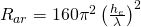# Electrically Small Antenna ERP

This page will (eventually) home a calculator for ERP, useful to those experimenting with 136 kHz, 472 kHz and 501 kHz amateur bands.

The calculator can now be found here: Electrically Short Antenna ERP Calculator.

The term electrically small is used to describe an antenna whose largest dimension, when measured from the input terminals, does not exceed one-eighth of the wavelength. Martin C, Carter P, Low Frequency Antennas, RCA Laboratories

“The most important factors affecting the antenna efï¬ciency are the wire resistance, the insulator equivalent loss resistance and the ground plane equivalent loss resistance. These factors are responsible of the antenna efï¬ciency, because they dissipate part of the antenna input power and, for this reason, the antenna gain can be much smaller than the
antenna directivity.” Trainotti V, Dorado L, Short Low and Medium Frequency Antenna Performance, Antennas and Propagation Magazine, IEEE (2005)

As I write this page, I regularly come across mention of the mathematics for VLF, LF and MF antenna design being un-coordinated and incomplete. This certainly seemed to be reflected in my research too! Many of the texts I read state sentences such as “Because of the general complexity of low-frequency antennas these effects cannot be rigorously calculated and it is necessary to determine them by approximation or experimentation.”

Look here and here Electrically Small Antenna Design for Low Frequency Systems REFERENCE ME PROPERLY!

# Things To Note

Just a few key points to note:

• The quality of an electrically small antenna, with regard to efficiency, power capability and bandwidth, increases with the antenna height.
• Top loading a vertical (umbrella, Marconi tee, inverted-L) increases the bandwidth by decreasing the reactance and the derivative of reactance with respect to frequency.
• Umbrella loading has the effect of reducing the feed voltage to the vertical and hence reduces power loss due to the base insulator.
• Model electrically small antennas using electrostatics and not electromagnetics.
• The effective height (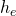) of a electrically small antenna is approximately half it’s actual height.

# Maths & Formula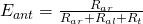where

•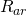is the radiation resistance
•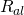is the loss resistance
•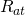is the resistance of the tuning element

Input reactance,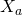, assuming the antenna is capacitive (i.e. electrically short, length shorter than quarter wavelength) is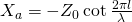Ohms
where

•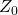is the characteristic impedance
•is length of an equivalent uniform transmission line
•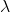is wavelength

If, in the special case, the radiator is a vertical cylinder, thenis equal to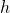, the height of the cylinder.

The characteristic impedance,, is calculated by one of the following formulas:

 Theoretical Experimental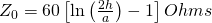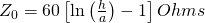Antenna input resistance,, is